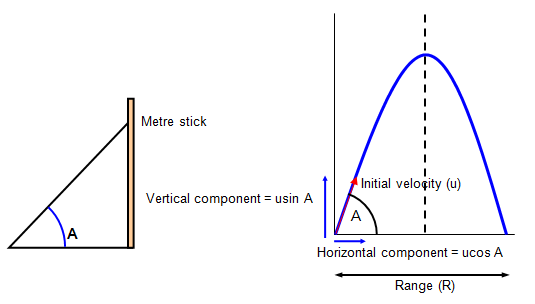# Slingshot and velocity

## Question:

How can you determine the velocity of a rock using only a slingshot, the rock and a metre stick. This is a freshman physics level question.

## Answer:

I am not too clear what you think of as a slingshot but I will imagine first that it is an elastic device like a catapult not that it really matters here. However it is important that I assume that you are given g.

Project the rock at a series of different angles and measure the range of the projectile path for each angle.The equation for the range of a projectile is:

Range = u2sin 2A/g where A is the angle of projection (measured with the horizontal) and u the velcoity of projection.

Now you are not given a protrcator so you can't measure the angle, however if you do it for a series of angles the one that gives the grearest range is when A = 45o and so 2A = 90o and so sin2A = 1.

This maximum range is therefoire: Rmax = u2/g from this the velocity of the rock can be found.

You could also plot a graph of range against angle to check the maximum range. Use the metre stick to get an idea of the angle by placing it vertically and sighting up towards it as you project the rock

A VERSION IN WORD IS AVAILABLE ON THE SCHOOLPHYSICS USB

© Keith Gibbs 2020